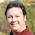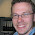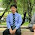## 18 May 2016

### Rounding amounts, divide cents over multiple lines

In previous articles I wrote about dealing with a missing cent when you need to divide a certain amount over multiple lines. In these articles, links are at the bottom, I described a method to calculate the difference on the last row.
Then a question arose (as a comment):
What if for example i have 42 records and i wish to divide 100 by 42. I would get a rounded value of 2.38. If i multiply this by 42 it would amount to just 99.96. What if want to spread the .04 difference on 4 records, not just the last record. In effect i'll be having 4 records with 2.39. Right now i'm doing this via cursor. Im kinda hoping i can do this using sql or analytic functions
Let's create a table first, called T:
```create table t
as
select rownum id
from dual
connect by level <= 42
```
In order to determine the number of rows, we need three pieces of information:
1. The amount that we need to divide
2. The total number of rows in the set
3. The difference between the rounded amount and the amount that we need to divide
``` select id
,100 amount
,count(*) over () entries
,round (100 / count(*) over (), 2) rounded
from t
```
To calculate how many rows will have the extra cent added or subtracted, the following formula is used.
```abs (amount - (entries * rounded)) * 100
```
When you want the rounding done on the "first" rows, a simple CASE expression can be used to mark the rows
```case
when rownum <= abs ((amount - (entries * rounded)) * 100)
then 'x'
end as indicator
```
The query now looks like the following, with the first ten rows:
```with amounts
as
( select id
,100 amount
,count(*) over () entries
,round (100 / count(*) over (), 2) rounded
from t
)
select id
,amount
,entries
,rounded
,case
when rownum <= abs ((amount - (entries * rounded)) * 100)
then 'x'
end as indicator
from amounts
;
ID     AMOUNT  ENTRIES    ROUNDED F
---------- ---------- ---------- ---------- -
1   100       42       2.38 x
2   100       42       2.38 x
3   100       42       2.38 x
4   100       42       2.38 x
5   100       42       2.38
6   100       42       2.38
7   100       42       2.38
8   100       42       2.38
9   100       42       2.38
10   100       42       2.38
```
The last piece of the puzzle is to determine if we need to add or subtract the cent. Using the SIGN function is an easy way to determine this.
```sign (amount - (entries * rounded)) as pos_neg
```
Putting everything together will give you the following query (with the first 10 rows)
```with amounts
as
( select id
,100 amount
,count(*) over () entries
,round (100 / count(*) over (), 2) rounded
from t
)
,indicators as
(
select id
,amount
,entries
,rounded
,case
when rownum <= abs ((amount - (entries * rounded)) * 100)
then 'x'
end as indicator
,sign (amount - (entries * rounded)) as pos_neg
from amounts
)
select id
,rounded +
(nvl2 (indicator, 0.01, 0) * pos_neg) final_amount
from indicators
;
ID FINAL_AMOUNT
---------- ------------
1    2.39
2    2.39
3    2.39
4    2.39
5    2.38
6    2.38
7    2.38
8    2.38
9    2.38
10    2.38
```

And there it is. The rounding is divided over the first four rows.

Don't want to use the first rows, but the last rows instead? Use the following expression to set the indicator
```case
when rownum >
case sign ((amount - (entries * rounded)))
when -1 then entries - abs ((amount - (entries * rounded)) * 100)
else entries - (amount - (entries * rounded)) * 100
end
then 'x'
end as indicator
```

## Links

#### 3 comments:

1.Instead of rounding how about using TRUNC(amount/count(*) over (),2), then you don't need to worry about the SIGN, instead you always add since (entries * rounded) will always be <= amount.

Next for the indicator column if you return either 0.01 or 0 instead of 'x' and null respectively, then you can just add the indicator column to the rounded column to get the final amount instead of using the NVL2 function.

1.Not sure how you mean the replacement of the round function? Could you perhaps show a small example? (or email it to me)
Yes, instead of the indicator it would be a bit shorter to do the calculation directly; Good point.

2.Thanks Alex, ill try this out.